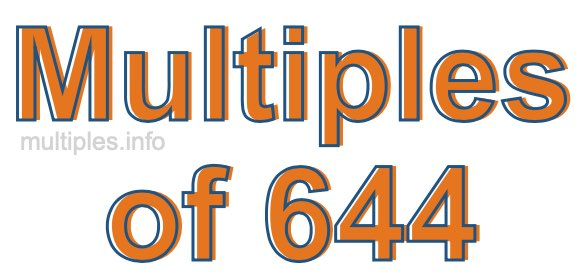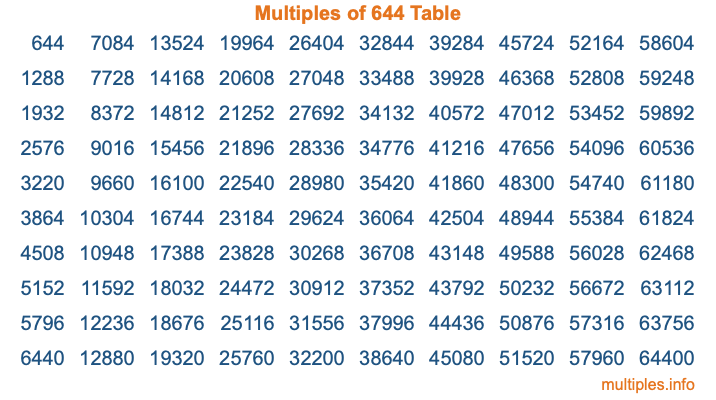Multiples of 644Welcome to the Multiples of 644 page. Here we will first teach you everything you will ever need to know about the multiples of 644, and then give you a study guide summary of everything we taught you to make sure you remember it all. Use this page to look up facts and learn information about the multiples of 644. This page will make you a multiples of six hundred forty-four expert!

Definition of Multiples of 644
Multiples of 644 are all the numbers that when divided by 644 equal an integer. Each of the multiples of 644 are called a multiple. A multiple of 644 is created by multiplying 644 by an integer.

Therefore, to create a list of multiples of 644, you start with 1 multiplied by 644, then 2 multiplied by 644, then 3 multiplied by 644, and so on for as long as you want. Thus, the list of the first five multiples of 644 is 644, 1288, 1932, 2576, and 3220. To see a larger list of multiples of 644, see the printable image of Multiples of 644 further down on this page. We also have a category where you can choose any nth multiple of 644.

Multiples of 644 Checker
The Multiples of 644 Checker below checks to see if any number of your choice is a multiple of 644. In other words, it checks to see if there is any number (integer) that when multiplied by 644 will equal your number. To do that, we divide your number by 644. If the the quotient is an integer, then your number is a multiple of 644.

Is  a multiple of 644?

Least Common Multiple of 644 and ...
A Least Common Multiple (LCM) is the lowest multiple that two or more numbers have in common. This is also called the smallest common multiple or lowest common multiple and is useful to know when you are adding our subtracting fractions. Enter one or more numbers below (644 is already entered) to find the LCM.

Check out our LCM Calculator if you need more details about the Least Common Multiple or if you need the LCM for different numbers for adding and subtraction fractions.

nth Multiple of 644
As we stated above, 644 is the first multiple of 644, 1288 is the second multiple of 644, 1932 is the third multiple of 644, and so on. Enter a number below to find the nth multiple of 644.

th multiple of 644

Multiples of 644 vs Factors of 644
644 is a multiple of 644 and a factor of 644, but that is where the similarities end. All postive multiples of 644 are 644 or greater than 644. All positive factors of 644 are 644 or less than 644.

Below is the beginning list of multiples of 644 and the factors of 644 so you can compare:

Multiples of 644: 644, 1288, 1932, 2576, 3220, etc.

Factors of 644: 1, 2, 4, 7, 14, 23, 28, 46, 92, 161, 322, 644

As you can see, the multiples of 644 are all the numbers that you can divide by 644 to get a whole number. The factors of 644, on the other hand, are all the whole numbers that you can multiply by another whole number to get 644.

It's also interesting to note that if a number (x) is a factor of 644, then 644 will also be a multiple of that number (x).

Multiples of 644 vs Divisors of 644
The divisors of 644 are all the integers that 644 can be divided by evenly. Below is a list of the divisors of 644.

Divisors of 644: 1, 2, 4, 7, 14, 23, 28, 46, 92, 161, 322, 644

The interesting thing to note here is that if you take any multiple of 644 and divide it by a divisor of 644, you will see that the quotient is an integer.

Multiples of 644 Table
Below is an image of the first 100 multiples of 644 in a table. The table is in chronological order, column by column. The first column has the first ten multiples of 644, the second column has the next ten multiples of 644, and so on.The Multiples of 644 Table is also referred to as the 644 Times Table or Times Table of 644. You are welcome to print out our table for your studies.

Negative Multiples of 644
Although not often discussed or needed in math, it is worth mentioning that you can make a list of negative multiples of 644 by multiplying 644 by -1, then by -2, then by -3, and so on, to get the following list of negative multiples of 644:

-644, -1288, -1932, -2576, -3220, etc.

Multiples of 644 Summary
Below is a summary of important Multiples of 644 facts that we have discussed on this page. To retain the knowledge on this page, we recommend that you read through the summary and explain to yourself or a study partner why they hold true.

There are an infinite number of multiples of 644.

A multiple of 644 divided by 644 will equal a whole number.

644 divided by a factor of 644 equals a divisor of 644.

The nth multiple of 644 is n times 644.

The largest factor of 644 is equal to the first positive multiple of 644.

644 is a multiple of every factor of 644.

644 is a multiple of 644.

A multiple of 644 divided by a divisor of 644 equals an integer.

644 divided by a divisor of 644 equals a factor of 644.

Any integer times 644 will equal a multiple of 644.

Multiples of a Number
Here you can get the multiples of another number, all with the same attention to detail as we did for multiples of 644 on this page.

Multiples of
Multiples of 645
Did you find our page about multiples of six hundred forty-four educational? Do you want more knowledge? Check out the multiples of the next number on our list!